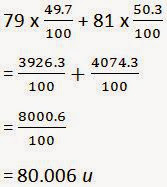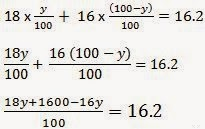# TEXTBOOK ANSWERS AND SOLUTIONS OF CBSE CLASS IX SCIENCE Chapter 4 Structure Of The Atom

1) Compare the properties of electrons, protons and neutrons.
ANSWER:-Electrons- Electrons are Negatively charged particles Protons-Protons are Positively charged particles. Neutron-Neutrons do not carry any charge and are neutral

2) What are the limitations of J.J. Thomson's model of the atom?
ANSWER:-The limitations of J.J. Thomson's model of the atom are: It could not explain the result of scattering experiment performed by Rutherford.
It did not have any experiment support.

3) What are the limitations of Rutherford's model of the atom?
ANSWER;-The limitations of Rutherford's model of the atom are It failed to explain the stability of an atom.
It doesn't explain the spectrum of hydrogen and other atoms.

4) Describe Bohr's model of the atom.
ANSWER:- The atom consists of a small positively charged nucleus at its center.
The whole mass of the atom is concentrated at the nucleus and the volume of the nucleus is much smaller than the volume of the atom.
All the protons and neutrons of the atom are contained in the nucleus.
Only certain orbits known as discrete orbits of electrons are allowed inside the atom.
while revolving in these discrete orbits electrons do not radiate energy. These orbits or cells are represented by the letters K, L, M, N etc.

6) Summarize the rules for writing of distribution of electrons in various shells for the first eighteen elements.
ANSWER:- If n gives the number of orbit or energy level, then 2n 2 gives the maximum number of electrons possible in a given orbit or energy level. Thus, First orbit or K-shell will have 2 electrons, Second orbit or L-shell will have 8 electrons, Third orbit or M-shell will have 18 electrons.
If it is the outermost orbit, then it should have not more than 8 electrons.
There should be step-wise filling of electrons in different orbits, i.e., electrons are not accompanied in a given orbit if the earlier orbits or shells are incompletely filled.

7) Define valency by taking examples of silicon and oxygen.
ANSWER:-The valency of an element is determined by the number of valence electrons present in the atom of that element. Valency of Silicon: It has electronic configuration: 2, 8, 4 Thus, the valency of silicon is 4 as these electrons can be shared with others to complete octet.
Valency of Oxygen: It has electronic configuration: 2, 6
Thus, the valency of oxygen is 2 as it will gain 2 electrons to complete its octet.

8) Explain with examples (i) Atomic number, (ii) Mass number, (iii) Isotopes and (iv) Isobars. Give any two uses of isotopes.
ANSWER :-( i) Atomic number: The atomic number of an element is the total number of protons present in the atom of that element. For example, nitrogen has 7 protons in its atom. Thus, the atomic number of nitrogen is 7.
(ii) Mass number: The mass number of an element is the sum of the number of protons and neutrons present in the atom of that element. For example, the atom of boron has 5 protons and 6 neutrons.
So, the mass number of boron is 5 + 6 = 11.
(iii) Isotopes: These are atoms of the same element having the same atomic number, but different mass numbers.
(iv) Isobars: These are atoms having the same mass number, but different atomic numbers i.e., isobars are atoms of different elements having the same mass number.
Two uses of isotopes:
One isotope of uranium is used as a fuel in nuclear reactors.
One isotope of cobalt is used in the treatment of cancer.

9) Na + has completely filled K and L shells. Explain.
ANSWER:-The atomic number of sodium is 11. So, neutral sodium atom has 11 electrons and its electronic configuration is 2, 8, 1. But Na + has 10 electrons. Out of 10, K-shell contains 2 and L-shell 8 electrons respectively. Thus, Na + has completely filled K and L shells.

10). If bromine atom is available in the form of, say, two isotopes 79 / 35Br (49.7%) and 81 / 35Br (50.3%), calculate the average atomic mass of bromine atom.11) The average atomic mass of a sample of an element X is 16.2 u. What are the percentages of isotopes 16 / 8 X and 18 / 8 X in the sample?
ANSWER:-Let the percentage of isotope 18 / 8 X be y%. Thus, the percentage of isotope 16 / 8 X will be (100 - y) %.
Therefore,18y + 1600 - 16y = 1620
2y + 1600 = 1620
2y = 1620 - 1600
y= 10
Therefore, the percentage ofisotope 18 / 8 X is 10%.
And, the percentage of isotope
16 / 8 X is (100 - 10) % = 90%.

12) If Z = 3, what would be the valency of the element? Also, name the element.
ANSWER:-By Z = 3, we mean that the atomic number of the element is 3. Its electronic configuration is 2, 1. Hence, the valency of the element is 1 (since the outermost shell has only one electron).
Therefore, the element with Z = 3 is lithium.

13) Composition of the nuclei of two atomic species X and Y are given as under
X      Y
Protons = 6      6
Neutrons = 6.   8
Give the mass numbers of X and Y. What is the relation between the two species?
ANSWER:-Mass number of X = Number of protons + Number of neutrons
= 6 + 6
= 12
Mass number of Y = Number of protons + Number of neutrons
= 6 + 8
= 14
These two atomic species X and Y have the same atomic number, but different mass numbers. Hence, they are isotopes

14) For the following statements, write T for 'True' and F for 'False'.
(a) J.J. Thomson proposed that the nucleus of an atom contains only nucleons.
False
(b) A neutron is formed by an electron and a proton combining together. Therefore, it is neutral.
False
(c) The mass of an electron is about 1 / 2000times that of proton.
True
(d) An isotope of iodine is used for making tincture iodine, which is used as a medicine.
False

15) Rutherfords alpha-particle scattering experiment was responsible for the discovery of
(a) Atomic nucleus
(b) Electron
(c) Proton
(d) Neutron

16) Isotopes of an element have
(a) The same physical properties
(b) Different chemical properties
(c) Different number of neutrons
(d) Different atomic numbers

17) Number of valence electrons in Cl - ion are:
(a) 16
(b) 8
(c) 17
(d) 18

18) Which one of the following is a correct electronic configuration of sodium?
(a) 2, 8
(b) 8, 2, 1
(c) 2, 1, 8
(d) 2, 8, 1

19) Complete the following table. Atomic num. Mass num.  No of   Neutrons
9.                       19.                   10
16.                       32.                   16
12.                       24.                   12
1.                         2.                     1
1.                         1.                     0